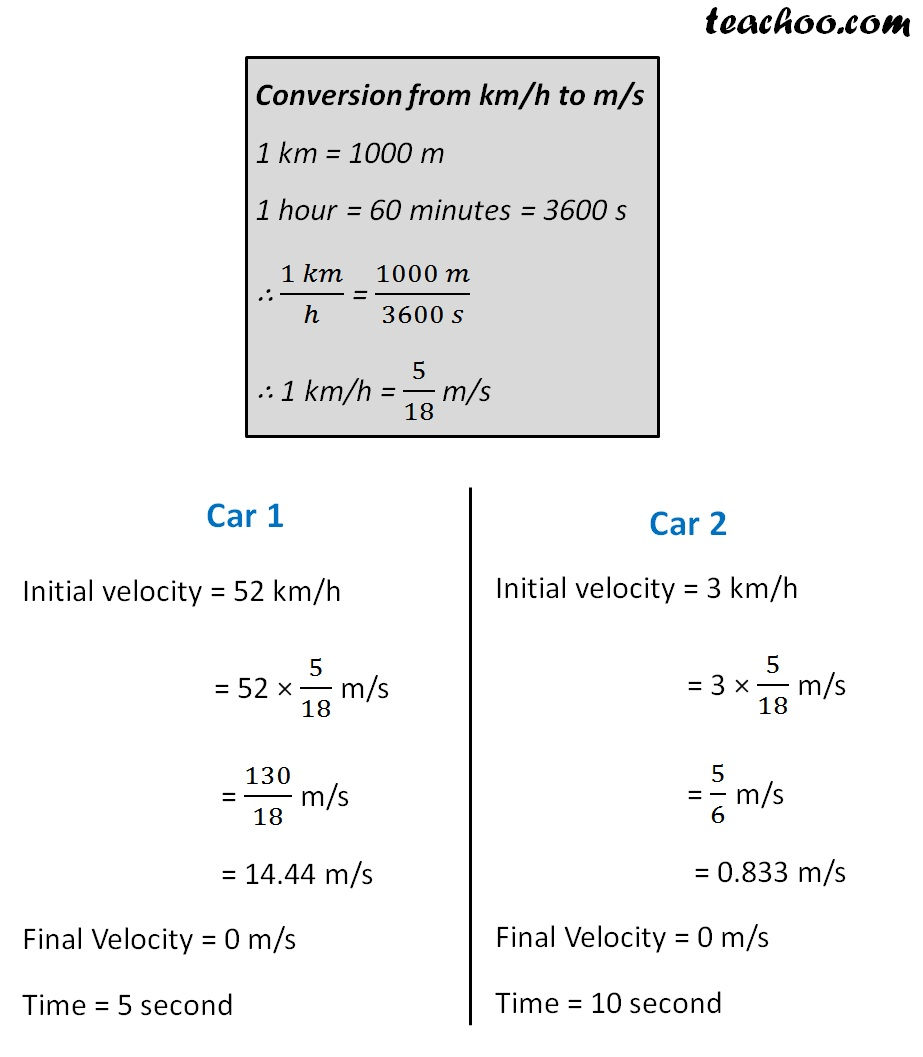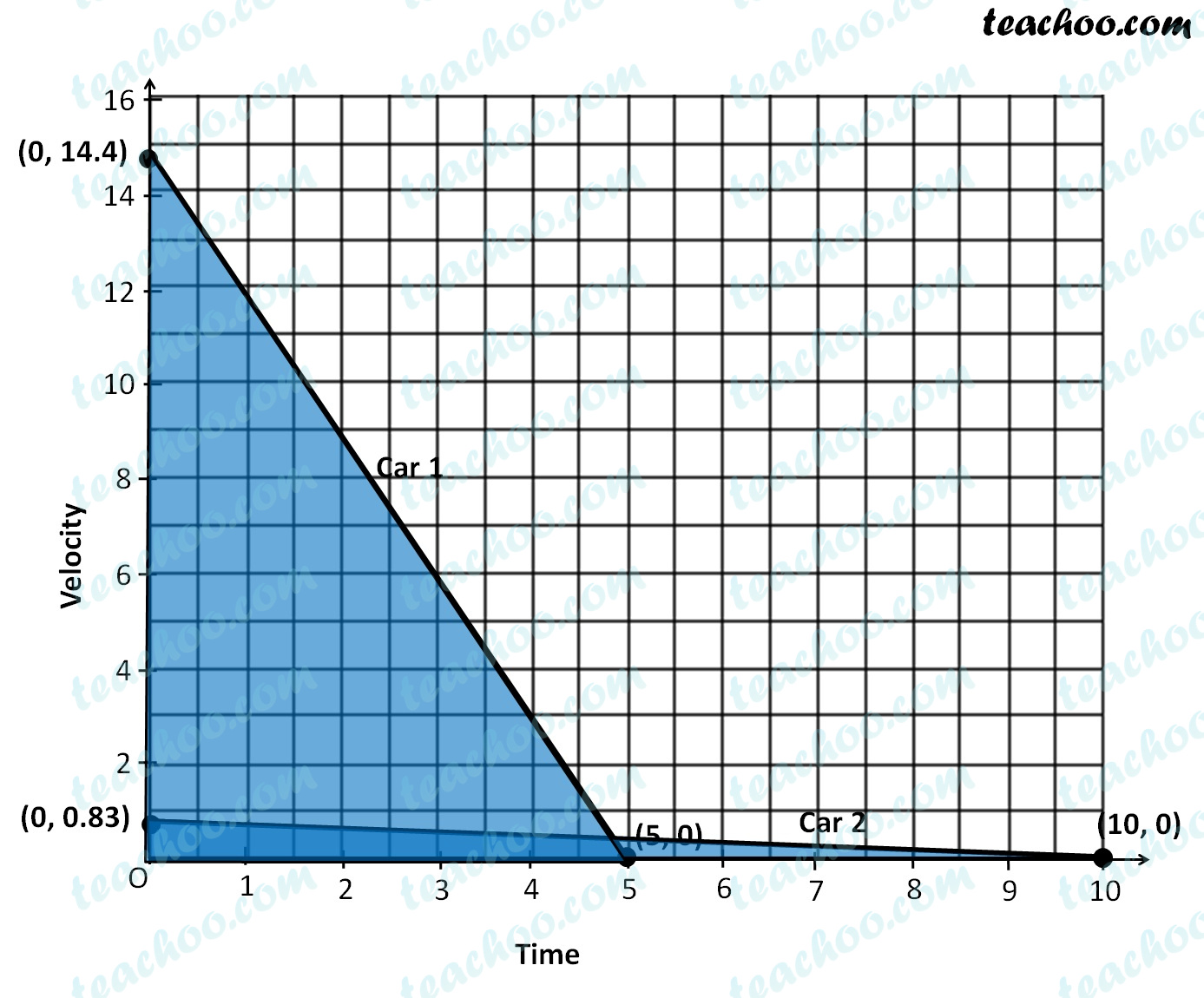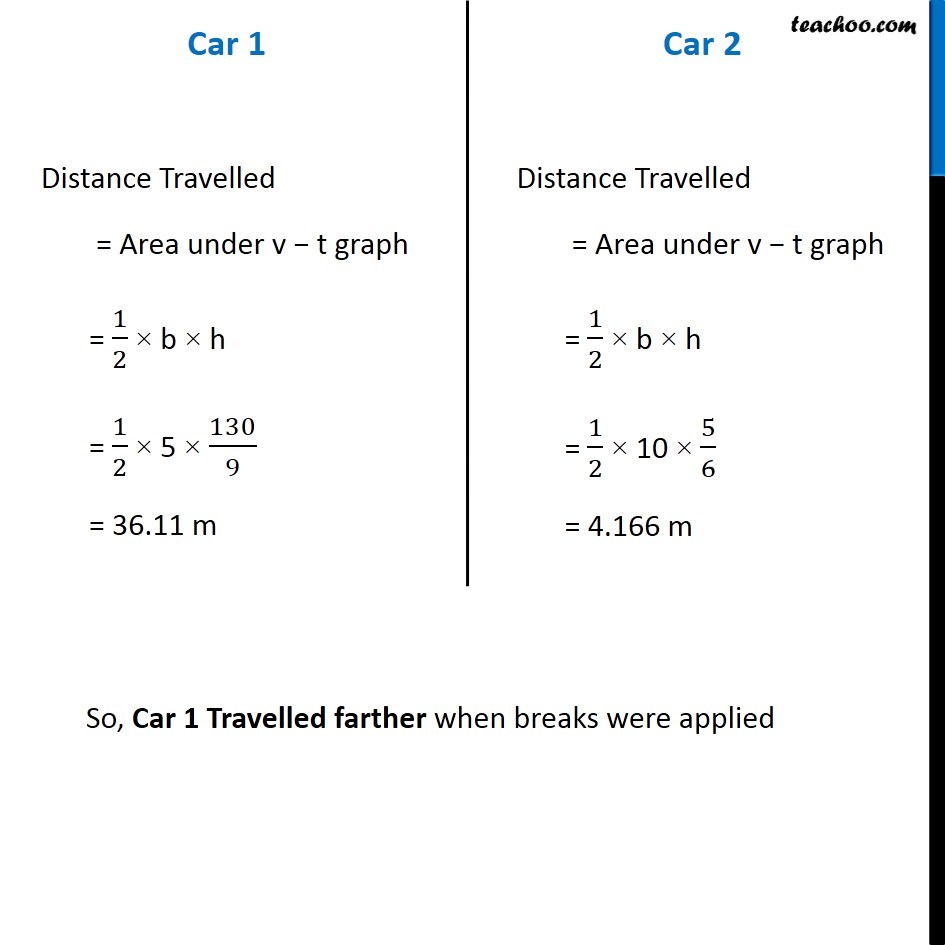NCERT Questions

Class 9
Chapter 8 Class 9 - Motion

## A driver of a car travelling at 52 km h–1 applies the brakes and accelerates uniformly in the opposite direction. The car stops in 5 s. Another driver going at 3 km h–1 in another car applies his brakes slowly and stops in 10 s. On the same graph paper, plot the speed versus time graphs for the two cars. Which of the two cars travelled farther after the brakes were applied?

First, let us convert both speeds in m/sLearn in your speed, with individual attention - Teachoo Maths 1-on-1 Class

### Transcript

Conversion from km/h to m/s 1 km = 1000 m 1 hour = 60 minutes = 3600 s ∴ (1 𝑘𝑚)/ℎ = (1000 𝑚)/(3600 𝑠) ∴ 1 km/h = 5/18 m/s Car 1 Initial velocity = 52 km/h = 52 × 5/18 m/s = 130/18 m/s = 14.44 m/s Final Velocity = 0 m/s Time = 5 second Car 2 Initial velocity = 3 km/h = 3 × 5/18 m/s = 5/6 m/s = 0.833 m/s Final Velocity = 0 m/s Time = 10 second Car 1 Distance Travelled = Area under v − t graph = 1/2 × b × h = 1/2 × 5 × 130/9 = 36.11 m Car 2 Distance Travelled = Area under v − t graph = 1/2 × b × h = 1/2 × 10 × 5/6 = 4.166 m So, Car 1 Travelled farther when breaks were applied